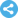American Journal of Mathematical and Computational Sciences
Manuscript Information

Lie Symmetries and Invariant-Solutions of the Potential Korteweg-De Vries Equation
American Journal of Mathematical and Computational Sciences
Vol.5 , No. 2, Publication Date: Aug. 5, 2020, Page: 9-16
405 Views Since August 5, 2020, 108 Downloads Since Aug. 5, 2020Paper in PDF (323K)Follow on

Authors

  Faya Doumbo Kamano, Department of Research, Distance Learning High Institute, Conakry, Guinea.  Bakary Manga, Department of Mathematics and Computer Sciences, University of Cheikh Anta Diop, Dakar, Senegal.  Joel Tossa, Institute of Mathematics and Physical Sciences, University of Abomey-Calavi, Porto-Novo, Benin.  Momo Bangoura, Department of Mathematics, University of Gamal Abdel Nasser, Conakry, Guinea.

Abstract

The purpose of this paper is to investigate the nonlinear partial differential equation, known as potential Korteweg-de Vries (p-KdV) equation. We have implemented the Harrison technique that makes use of differential forms and Lie derivatives as tools to find the point symmetry algebra for the p-KdV equation. This approach allows us to obtain five infinitesimal generators of point symmetries. Fixing each generator of symmetries that we have found, we construct a complete set of functionally independent invariants, corresponding to the new independent and dependent variables. Using these new variables, called “similarity variables”, the reduced equations have been constructed systematically, which leads to exact solutions that are group-invariant solutions for the p-KdV equation. The obtained solutions are of two types. The reduced equations from the generator of space and time translation groups are the first and the third order ordinary differential equations respectively and lead to the Travelling-invariant solutions. Then, the reduced equation from the generator of the Galilean boosts is the first order ordinary differential equation and leads to Galilean-invariant solutions. Under the generator of scaling symmetries, the potential KdV equation reduces to the third order ordinary differential equation, which does not admit symmetries. And then, there are no functionally independent invariants for that last equation, its solutions are essentially new functions not expressible in terms of standard special functions.

Keywords

Symmetries, Differential Forms, Lie Derivative, Korteweg-de Vries Equations, Invariant Solutions

Reference

  Abdul-Majid Wazwaz, Partial Differential Equations and Solitary Waves Theory, Nonlinear Physical Sciences, Springer, USA, 2009.  B. Kent Harrison, F. B. Estabrook, Geometric Approach to Invariance Groups and Solution of Partial Differential Systems, J. Math. Phys., 1971, V. 12, 653-666.  B. Kent Harrison, The differential Form Method for Finding Symmetries, SIGMA, Vol. 1 (2005), Paper 001, 12 pages.  B. Kent Harrison, Differential Form Symmetry Analysis of Two Equations Cited by Fushchych, Department of Physics and Astronomy, Brigham Young University, Provo, Utah 84602 U.S.A,, V. 1, 21-33 (1997).  Biswas, A., Kumar, S., Krishnan, E. V., Ahmed, B., Strong, A., Johnson, S., Yildirim, A., Topological solitons and other solutions to potential KdV equation. Rom. Rep. Phys. 65 (4), 1125-1137 (2013).  C. A. G Ìomez Sierra, M. Molati, M. P. Ramollo, Exact Solutions of a Generalized KdV-mKdV Equation, International Journal of Nonlinear Science, Vol. 13 (2012) No. 1, pp. 94-98.  El-Wakil, S. A., Abdou, M. A., Elhanbaly, A., solitons and periodic wave solutions for nonlinear evolution equations. Phys. Lett. A 353 (1), 40â47 (2006).  G. W. Bluman, and S. Kumei, Symmetries and Differential Equations, Springer Verlage, New York, 1989.  Guo, Y., Wang, Y., Weierstrass elliptic function solu- tions for a (N+1) dimensional potential KdV equation. Appl. Math. Comput. 217 (20), 8080â8092 (2011).  Houria Triki, Turgut Ak, Mehmet Ekici, Abdullah Sonmezoglu, Mohammad Mirzazadeh, Abdul Hamid Kara, Tugba Aydemir, Some new exact wave solutions and conservation laws of potential Kortewegâde Vries equation Nonlinear dynamic DOI 10.1007/s11071-017-3467-4, 2017.  Mehdi Nadjafikhah and Seyed-Reza Hejazi, Lie Symmetries and Solutions of KdV Equation, Departmant of Mathematics, Iran University of Science and Technology, Narmak, Tehran, Iran, International Mathematial Forum, 4 (4), 165-176 (2009).  P. J. Olver, Applications of Lie Groups to Differential Equations, Second Edition, GTM, Vol. 107, Springer Verlage, New York, 1993.  Wang, G.-W., Xu, T.-Z., Ebadi, G., Johnson, S., Strong, A. J., Biswas, A., solitons, shock waves, and other solutions to potential KdV equation. Nonlinear Dyn. 76 (2), 1059â1068 (2014).  Wazwaz, A.-M., New solitary wave solutions to the modified Kawahara equation. Phys. Lett. A 360 (4â5), 588â592 (2007).